## Tuesday, April 29, 2014

Steepest gradient descent is an algorithm for optimizing quadratic forms. The idea is, given a wok-shaped convex surface, if you start at a certain point and each time you move in the direction of fastest descent, then, step by step, you will eventually get to the lowest point.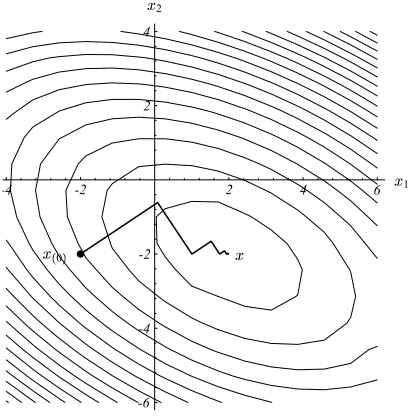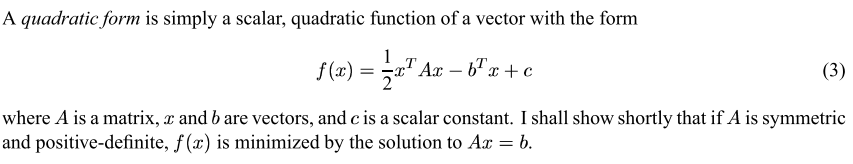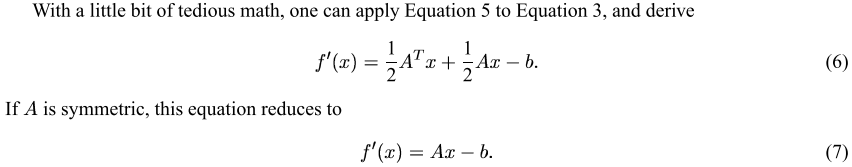$f'(x)$ is the gradient vector, i.e. it points to the direction of fastest ascent. We want the opposite, so let $r(x) = -f'(x) = b - Ax$.

You might ask, why not just invert A and solve the equation to get the optimal $x$? In simple cases this is indeed possible, but here we use an iterative approach for it's very useful in more general situations.

Back to steepest descent method. First we choose a random start point $x_{(0)}$, and descend in the direction of $r_{(0)}$, i.e.

\begin{align*} x_{(1)} &= x_{(0)} + \alpha r_{(0)} \\ &= x_{(0)} + \alpha (b - Ax_{(0)}) \\ \end{align*}

Everything is settled, except that we don't know $\alpha$, i.e. we known the step size to take. To maximize efficiency, we want to reach the lowest point possible in every step.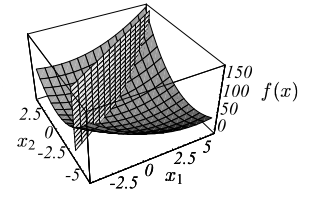This is solved this way: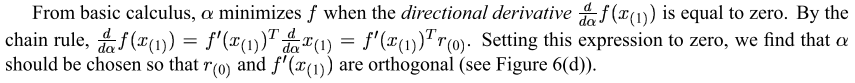Note that $\frac{d}{d\alpha}x_{(1)} =\frac{d}{d\alpha}(x_{(0)} + \alpha r_0) = r_0$.

This means the gradient at the next point should be perpendicular to the gradient at the current point! (Hence the zigzag in the first figure.) The calculation of $\alpha$ easily follows: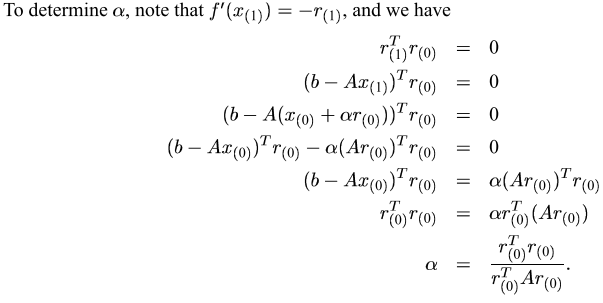Now all pieces of the puzzle are here, we can have the big picture: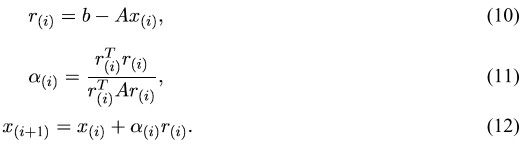The computational burden (in terms of matrix multiplication, which is the bulky part) can be reduced by half if you use a small trick: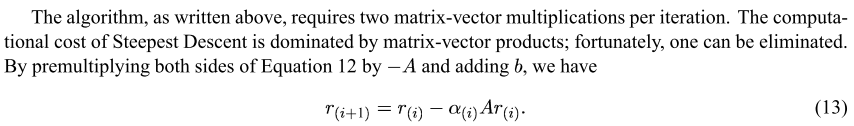So, here is the first few iterations of the algorithm:

\begin{align*} \hline \\ r_{(0)} &= b - Ax_{(0)} \\ \alpha_{(0)} &= \frac{r_{(0)}^T r_{(0)}}{r_{(0)}^T A r_{(0)}} \\ x_{(1)} &= x_{(0)} + \alpha_{(0)} r_{(0)} \\ \hline \\ r_{(1)} &= r_{(0)} - \alpha_{0}Ar_{(0)} \\ \alpha_{(1)} &= \frac{r_{(1)}^T r_{(1)}}{r_{(1)}^T A r_{(1)}} \\ x_{(2)} &= x_{(1)} + \alpha_{(1)} r_{(1)} \\ \hline \\ r_{(2)} &= r_{(1)} - \alpha_{1}Ar_{(1)} \\ \alpha_{(2)} &= \frac{r_{(2)}^T r_{(2)}}{r_{(2)}^T A r_{(2)}} \\ x_{(3)} &= x_{(2)} + \alpha_{(2)} r_{(2)} \\ \hline \\ \vdots \end{align*}

The order of calculation and reuse of variables is illustrated here: